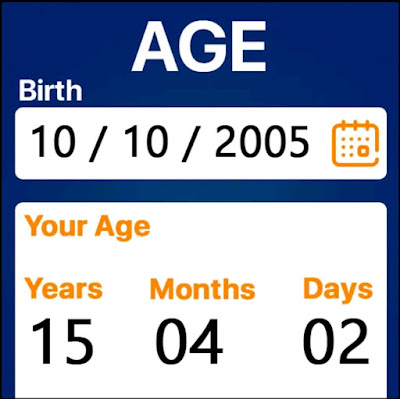Age Calculator app for Android Calculate your age anywhere, anytime - Technology Rom -->

Age Calculator app for Android Calculate your age anywhere, anytime

Calc Master - The most popular age calculator. Calculate your age anywhere, anytime

Welcome to Calc Master - Age Calculator. Calculate your age from anywhere, anytime.

Calc Master - Age Calculator App by Oogway App is one of the best age calculator apps. If you want to know your total age, years, months, days, hours, minutes and seconds then download this age calculator app now. Calc Master is not just an age calculator app but helps you to calculate pet age, GST taxation, units, math calculations etc.

Want to calculate your age? Or someone's age? Then don't worry! Here is the new Cal Master Age calculator App. You can calculate age or date of birth in seconds from anywhere anytime. You don't have to spend minutes on it. Get fast results! Make Age Calculator your friend and don't worry about calculating your age!

Gone are the days of calculating your age by counting on your fingers. Now a new and amazing Age Calculator app has come to make it easier for you. Now age calculation is a matter of seconds!

You can use this age calculator completely free! You don't have to spend a single penny on it and still get 100% accurate results in seconds. Just enter the correct data and quickly calculate the age. You would never see such a fast and accurate age calculator app that is absolutely easy and free.

Tutorial

1. Open the Calc master age calculator application

2. You will see a lot of options, you can choose according to your need. There will be options to help you calculate pet age calculators, calculate unts, loan EMI, GST and more. Select the age calculator option.

3. Now you know whose age you want to calculate. If you want to calculate your age, enter all the required accurate data without errors to get an accurate result. If you want to calculate your friend's age, enter his details correctly. Likewise, enter the details of each age you want to calculate.

4. Finally! Get accurate results in seconds.

Function

1. Pet Age Calculator: This calculator allows you to calculate the age of your pet or your friend's pet, basically any pet.

2. EMI Calculator: Age Calculator app also helps you to calculate EMI.

3. GST Calculator: Want to calculate GST taxes, this is the app you should count on.

4. Units Calculator: Calculate units, convert degrees Celsius, Kelvin, Fahrenheit, millimeters, meters, kilometers, etc.

5. Calculator: Use our simple calculator to calculate any math number.

This is how it works! Easy to use and understand. Make it your best friend. Built with the best user interface, features and more, Age Calculator is made with every age group in mind so anyone can use it easily! Try the Age Calculator yourself to calculate your age and you will know exactly how it works! Once installed, you will never delete the application from your mobile phone and have it with you forever.# Age Calculator app for Android Calculate your age anywhere, anytime

Age Calculator app for Android Calculate your age anywhere, anytime

Calc Master - The most popular age calculator. Calculate your age anywhere, anytime

Welcome to Calc Master - Age Calculator. Calculate your age from anywhere, anytime.

Calc Master - Age Calculator App by Oogway App is one of the best age calculator apps. If you want to know your total age, years, months, days, hours, minutes and seconds then download this age calculator app now. Calc Master is not just an age calculator app but helps you to calculate pet age, GST taxation, units, math calculations etc.

Want to calculate your age? Or someone's age? Then don't worry! Here is the new Cal Master Age calculator App. You can calculate age or date of birth in seconds from anywhere anytime. You don't have to spend minutes on it. Get fast results! Make Age Calculator your friend and don't worry about calculating your age!

Gone are the days of calculating your age by counting on your fingers. Now a new and amazing Age Calculator app has come to make it easier for you. Now age calculation is a matter of seconds!

You can use this age calculator completely free! You don't have to spend a single penny on it and still get 100% accurate results in seconds. Just enter the correct data and quickly calculate the age. You would never see such a fast and accurate age calculator app that is absolutely easy and free.

Tutorial

1. Open the Calc master age calculator application

2. You will see a lot of options, you can choose according to your need. There will be options to help you calculate pet age calculators, calculate unts, loan EMI, GST and more. Select the age calculator option.

3. Now you know whose age you want to calculate. If you want to calculate your age, enter all the required accurate data without errors to get an accurate result. If you want to calculate your friend's age, enter his details correctly. Likewise, enter the details of each age you want to calculate.

4. Finally! Get accurate results in seconds.

Function

1. Pet Age Calculator: This calculator allows you to calculate the age of your pet or your friend's pet, basically any pet.

2. EMI Calculator: Age Calculator app also helps you to calculate EMI.

3. GST Calculator: Want to calculate GST taxes, this is the app you should count on.

4. Units Calculator: Calculate units, convert degrees Celsius, Kelvin, Fahrenheit, millimeters, meters, kilometers, etc.

5. Calculator: Use our simple calculator to calculate any math number.